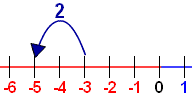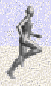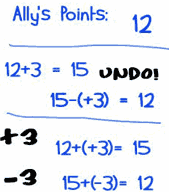# How to Add and Subtract Positive and Negative Numbers

## Numbers Can be Positive or Negative

This is the Number Line:Negative Numbers (−) Positive Numbers (+)"−" is the negative sign. "+" is the positive sign

## No Sign Means Positive

If a number has no sign it usually means that it is a positive number.

Example: 5 is really +5

## Play with it!

On the Number Line positive goes to the right and negative to the left.

Try the sliders below and see what happens:

## Balloons and Weights

Let us think about numbers as balloons (positive) and weights (negative):This basket has balloons and weights tied to it:

• The balloons pull up (positive)
• And the weights drag down (negative)the basket gets pulled upwards (positive)

### Example: 2 + 3 = 5

is really saying

"Positive 2 plus Positive 3 equals Positive 5"

We could write it as (+2) + (+3) = (+5)

## Subtracting A Positive Number

Subtracting positive numbers is just simple subtraction.We can take away balloons (we are subtracting positive value)

the basket gets pulled downwards (negative)

### Example: 6 − 3 = 3

is really saying

"Positive 6 minus Positive 3 equals Positive 3"

We could write it as (+6) − (+3) = (+3)

Now let's see what adding and subtracting negative numbers looks like:the basket gets pulled downwards (negative)

### Example: 6 + (−3) = 3

is really saying

"Positive 6 plus Negative 3 equals Positive 3"

We could write it as (+6) + (−3) = (+3)

The last two examples showed us that taking away balloons (subtracting a positive) or adding weights (adding a negative) both make the basket go down.

So these have the same result:

• (+6) − (+3) = (+3)
• (+6) + (−3) = (+3)

In other words subtracting a positive is the same as adding a negative.

## Subtracting A Negative NumberLastly, we can take away weights (we are subtracting negative values)

the basket gets pulled upwards (positive)

### Example: What is 6 − (−3) ?

6−(−3) = 6 + 3 = 9

Yes indeed! Subtracting a Negative is the same as adding!

Two Negatives make a Positive

## What Did We Find?### Positive and Negative Together ...Subtracting a Positive
or
is
Subtraction

6−(+3) = 6 3 = 3

### Example: What is 5 + (−7) ?

5+(−7) = 5 7 = −2

### Subtracting a negative ...Subtracting a Negative is the same as Adding

### Example: What is 14 − (−4) ?

14−(−4) = 14 + 4 = 18

## The Rules:

It can all be put into two rules:

Rule   Example +(+) Two like signs become a positive sign3+(+2) = 3 + 2 = 5 6−(−3) = 6 + 3 = 9 Two unlike signs become a negative sign7+(−2) = 7 − 2 = 5 8−(+2) = 8 − 2 = 6

They are "like signs" when they are like each other (in other words: the same).

So, all you have to remember is:

Two like signs become a positive sign

Two unlike signs become a negative sign

### Example: What is 5+(−2) ?

+(−) are unlike signs (they are not the same), so they become a negative sign.

5+(−2) = 5 2 = 3

### Example: What is 25−(−4) ?

−(−) are like signs, so they become a positive sign.

25−(−4) = 25+4 = 29

## Starting Negative

Using The Number Line can help:

### Example: What is −3+(+2) ?

+(+) are like signs, so they become a positive sign.

−3+(+2) = −3 + 2Start at −3 on the number line,
move forward 2 and you end up at −1

−3+(+2) = −3 + 2 = −1

### Example: What is −3+(−2) ?

+(−) are unlike signs, so they become a negative sign.

−3+(−2) = −3 2Start at −3 on the number line,
move back 2 and you end up at −5

−3+(−2) = −3 2 = −5

## Now Play With It!Try playing Casey Runner, you need to know the rules of positive and negative to succeed!

## A Common Sense Explanation

And there is a "common sense" explanation:If I say "Eat!" I am encouraging you to eat (positive)

If I say "Do not eat!" I am saying the opposite (negative).

Now if I say "Do NOT not eat!", I am saying I don't want you to starve, so I am back to saying "Eat!" (positive).

So, two negatives make a positive, and if that satisfies you, then you are done!

## Another Common Sense Explanation

A friend is +, an enemy is

 + + ⇒ + a friend of a friend is my friend + − ⇒ − a friend of an enemy is my enemy − + ⇒ − an enemy of a friend is my enemy − − ⇒ + an enemy of an enemy is my friend

## A Bank Example

### Example: Last year the bank subtracted \$10 from your account by mistake, and they want to fix it.

So the bank must take away a negative \$10.

Let's say your current balance is \$80, so you will then have:

\$80−(−\$10) = \$80 + \$10 = \$90

So you get \$10 more in your account.

## A Long Example You Might Like

### Ally's PointsAlly can be naughty or nice. So Ally's parents have said

"If you are nice we will add 3 points (+3).
If you are naughty, we take away 3 points (−3).
When you reach 30 Points you get a toy."

 Ally starts the day with 9 Points: 9 Ally's Mom discovers spilt milk: 9 − 3 = 6 Then Dad confesses he spilt the milk and writes "undo". How do we "undo" a minus 3? We add 3 back again! So Mom calculates: 6 − (−3) = 6 + 3 = 9So when we subtract a negative, we gain points
(i.e. the same as adding points).So Subtracting a Negative is the same as Adding

 A few days later. Ally has 12 points. Mom adds 3 points because Ally's room is clean. 12 + 3 = 15 Dad says "I cleaned that room" and writes "undo" on the chart. Mom calculates: 15 − (+3) = 12 Dad sees Ally brushing the dog. Writes "+3" on the chart. Mom calculates: 12 + (+3) = 15 Ally throws a stone against the window. Dad writes "−3" on the chart. Mom calculates: 15 + (−3) = 12See: both "15 − (+3)" and "15 + (−3)" result in 12.

So:

It doesn't matter if you subtract positive points
you still end up losing points.So Subtracting a Positive
or